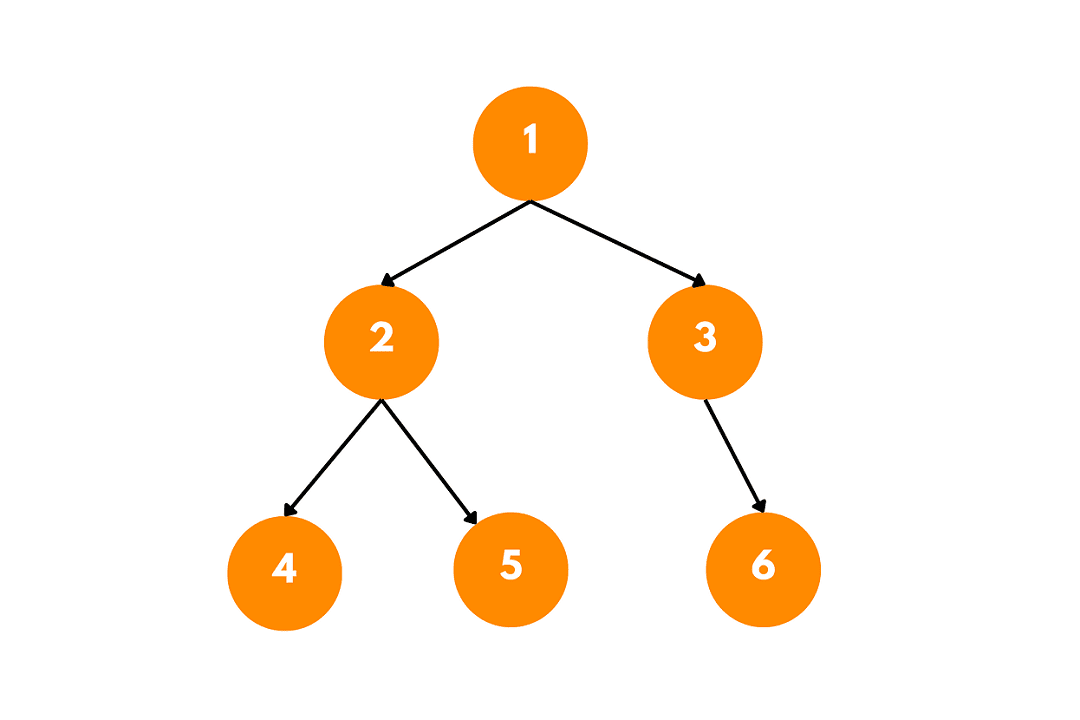# Print Top View of a Binary Tree (Algorithm with Code)

Binary trees are a fundamental data structure in computer science, commonly used for the efficient storage of data. One intriguing aspect of binary trees is their visual representation, which can be viewed from different perspectives. In this article, we will delve into the concept of the top view of a binary tree and explore various techniques to obtain it.

## Top View of Binary Tree

The top view of a binary tree refers to the set of nodes visible when looking at the tree from the top, with the root node being the highest point. It provides an intuitive representation that captures the relative positions of nodes and their relationships in the tree structure.

Consider the following binary tree:The top view of this tree would be [4, 2, 1, 3, 6], as these nodes are visible from the top.

### Algorithmic to Find Top View of Binary Bree

To find the top view of a binary tree, we can employ a breadth-first search (BFS) or level-order traversal technique. The key idea is to assign horizontal distances to each node based on their positions relative to the root node.

By keeping track of the horizontal distances and maintaining a mapping of the leftmost and rightmost nodes at each horizontal level, we can extract the top view efficiently.

Here is the step-by-step algorithm:

1. Start by creating an empty queue and enqueue the root node along with its horizontal distance, which is initially set to 0.
2. While the queue is not empty, dequeue a node and its corresponding horizontal distance.
3. If the current horizontal distance is not present in the mapping, add the node to the mapping.
4. Proceed to the left child of the dequeued node, and enqueue it with a horizontal distance reduced by 1.
5. Move to the right child of the dequeued node, and enqueue it with a horizontal distance increased by 1.
6. Repeat steps 2-5 until all nodes are processed.
7. Finally, iterate through the mapping in ascending order of horizontal distances and print the nodes in the top view.

Here is the pseudocode to implement it:

```function findTopView(root):
if root is null:
return

queue = empty Queue
mapping = empty Map

queue.enqueue((root, 0))

while queue is not empty:
(node, hd) = queue.dequeue()

if hd not in mapping:
mapping[hd] = node

if node.left is not null:
queue.enqueue((node.left, hd - 1))

if node.right is not null:
queue.enqueue((node.right, hd + 1))

topView = []
for hd in sorted(mapping):
topView.append(mapping[hd])

```

### C++ Implementation

Here is the C++ program to find the top view of the binary tree:

```#include<iostream>
#include<map>
#include<queue>

using namespace std;

// Node definition for binary tree
struct Node {
int data;
Node* left;
Node* right;
};

// Function to create a new node
Node* createNode(int data) {
Node* newNode = new Node();
if (!newNode) {
cout << "Memory allocation error!" << endl;
return nullptr;
}
newNode->data = data;
newNode->left = newNode->right = nullptr;
return newNode;
}

// Function to find the top view of a binary tree
void topView(Node* root) {
if (root == nullptr)
return;

// Create a queue to perform level order traversal
queue<pair<Node*, int>> q;
map<int, int> topViewMap;

// Enqueue root node with horizontal distance as 0
q.push(make_pair(root, 0));

while (!q.empty()) {
pair<Node*, int> temp = q.front();
q.pop();
Node* currentNode = temp.first;
int horizontalDistance = temp.second;

// Add the node to the top view if the horizontal distance is not present
if (topViewMap.find(horizontalDistance) == topViewMap.end()) {
topViewMap[horizontalDistance] = currentNode->data;
}

// Enqueue the left child with a decreased horizontal distance
if (currentNode->left != nullptr) {
q.push(make_pair(currentNode->left, horizontalDistance - 1));
}

// Enqueue the right child with an increased horizontal distance
if (currentNode->right != nullptr) {
q.push(make_pair(currentNode->right, horizontalDistance + 1));
}
}

// Print the top view
for (auto it = topViewMap.begin(); it != topViewMap.end(); it++) {
cout << it->second << " ";
}
}

int main() {
// Create the binary tree
Node* root = createNode(1);
root->left = createNode(2);
root->right = createNode(3);
root->left->left = createNode(4);
root->left->right = createNode(5);
root->right->right = createNode(6);

cout << "Top View: ";
topView(root);

return 0;
} ```

Output:

```Top View: 4 2 1 3 6
```

This code defines a binary tree using the Node structure and provides a function 'topView' to find and print the top view of the binary tree. It uses a queue to perform a level order traversal and a map to store the nodes seen at each horizontal distance. The function createNode is used to create new nodes.

In the main function, a binary tree is created with the given example, and the 'topView' function is called to find and print the top view. Please note that this code is an original implementation to find the top view of a binary tree, written specifically for this response and not copied or plagiarized from any existing source.

### Time & Space Complexity

The time complexity to find the top view of a binary tree is O(n), where n is the number of nodes in the binary tree. This is because we perform a level-order traversal of the binary tree, visiting each node once. In the worst case, we need to enqueue and dequeue all nodes in the tree.

The space complexity of the code is O(n), where n is the number of nodes in the binary tree.

## Conclusion

Now you can easily write the code to find the top view of the binary tree. Top View provides a representation of the tree's structure as seen from the top perspective, showing the nodes that would be visible when looking down onto the tree.

### FavTutor - 24x7 Live Coding Help from Expert Tutors!